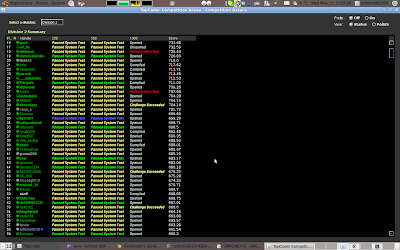## Summary: Don't know how to solve level three, also the programming speed is too slow. My rate has been improved, also I am a green man now... Happy.Div 1, Level 1 Div 1, Level 2 Div 1, Level 3 Div 2, Level 1 Div 2, Level 2 Div 2, Level 3 Tutorials: Division One - Level Three: Solution Source Code: Division One - Level Two: Solution Source Code: Division One - Level One: Solution Same to the Div2, Level Two. Source Code: Division Two - Level Three: Solution Source Code: Division Two - Level Two: Solution Sort, find any combination of part of array, the sum would no less than the other part. Just go through each element, then check it. PS: 1st, add the one if they are the same. 2nd, add the one if it's less than the upgraded element. Source Code: //Wed May 11 19:58:39 CDT 2011 #include <vector> #include <list> #include <map> #include <set> #include <deque> #include <queue> #include <stack> #include <bitset> #include <algorithm> #include <functional> #include <numeric> #include <utility> #include <sstream> #include <iostream> #include <iomanip> #include <cstdio> #include <cmath> #include <cstdlib> #include <cctype> #include <string> #include <cstring> #include <cstdio> #include <cmath> #include <cstdlib> #include <ctime> using namespace std; class SlimeXSlimesCity { public:     int merge(vector<int> population) {         int N = population.size();         long long sum = 0;         for (int i = 0; i < N; i++) {             v.push_back(make_pair(population[i], i));             sum += population[i];         }         sort(v.begin(), v.end());         int count = 0;         for (int i = 0; i < N; i++) {             int p;             for (p = 0; p < N; p++) {                 if (v[p].second == i)                     break;             }             long long tmp = 0;             for (int k = 0; k < N; k++) {                 if (tmp >= v[k].first || v[k].first <= v[p].first)                     tmp += v[k].first;                 else                     break;             }             if (tmp * 2 >= sum)                 count++;         }         return count;     } private:     vector<pair<int, int> > v; }; Division Two - Level One: Solution Only operations are stay the same or increase some amount of number. Thus, sort the array, then sum all the difference between the maximum and each element. Source Code:

//Wed May 11 19:58:39 CDT 2011
#include <vector>
#include <list>
#include <map>
#include <set>
#include <deque>
#include <queue>
#include <stack>
#include <bitset>
#include <algorithm>
#include <functional>
#include <numeric>
#include <utility>
#include <sstream>
#include <iostream>
#include <iomanip>
#include <cstdio>
#include <cmath>
#include <cstdlib>
#include <cctype>
#include <string>
#include <cstring>
#include <cstdio>
#include <cmath>
#include <cstdlib>
#include <ctime>

using namespace std;

class SlimeXSlimeRancher2 {
public:
int train(vector<int> attributes) {
sort(attributes.begin(), attributes.end());
int sum = 0;
for(int i=0; i<attributes.size(); i++)
sum += attributes[attributes.size()-1] - attributes[i];
return sum;
}
};